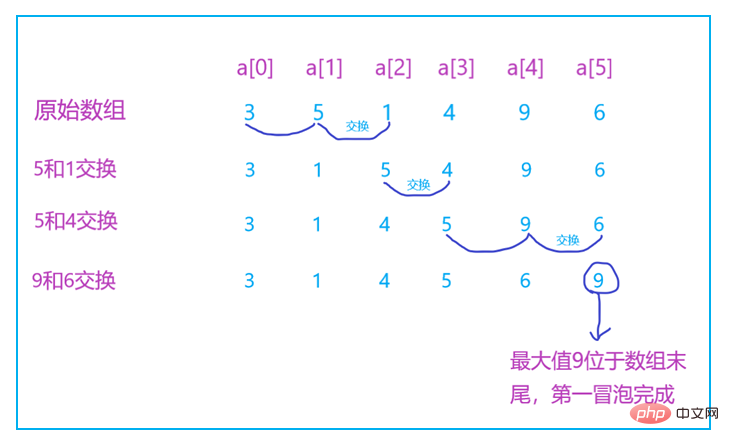# Java冒泡排序的详细介绍（代码示例）发布日期：2019-04-27  来源：QJ1gnK  作者：xinb2b.cn  浏览次数：821、 导言

• 冒泡排序、选择排序、插入排序，平均时间复杂度都是O(n2)
• 希尔排序、归并排序、快速排序、堆排序，平均时间复杂度都是O(nlogn)
• 计数排序、基数排序、桶排序，平均时间复杂度都是O(n + k)

1. 原地排序：指的是在排序的过程当中不会占用额外的存储空间，空间复杂度为O(1)。
2. 排序算法的稳定性：一个稳定的排序，指的是在排序之后，相同元素的前后顺序不会被改变，反之就称为不稳定。举个例子：一个数组 [3，5，1，4，9，6，6，12] 有两个6（为了区分，我把其中一个 6 加粗），如果排序之后是这样的：[1，3，4，5，6，6，9，12]（加粗的 6 仍然在前面），就说明这是一个稳定的排序算法。
2. 言归正传```public class BubbleSort {

//data表示整型数组，n表示数组大小
public static void bubbleSort(int[] data, int n){
//数组大小小于等于1，无须排序
if (n <= 1) return;

for (int i = 0; i < n; i++) {
for (int j = 0; j < n - i - 1; j++) {
//如果data[j] > data[j + 1]，交换两个数据的位置
if (data[j] > data[j + 1]){
int temp = data[j];
data[j] = data[j + 1];
data[j + 1] = temp;
}
}
}
}
}```

```public class BubbleSort {

//优化后的冒泡排序
//data表示整型数组，n表示数组大小
public static void bubbleSort(int[] data, int n){
//数组大小小于等于1，无须排序，返回空
if (n <= 1) return;
for (int i = 0; i < n; i++) {
boolean flag = false;//判断是否有数据交换

for (int j = 0; j < n - i - 1; j++) {
//如果data[j] > data[j + 1]，交换两个数据的位置
if (data[j] > data[j + 1]){
int temp = data[j];
data[j] = data[j + 1];
data[j + 1] = temp;

flag = true;//表示有数据交换
}
}
//如果没有数据交换，则直接退出循环
if (!flag) break;
}
}
}```

1. 冒泡排序是基于数据比较的
2. 最好情况时间复杂度是O(n)，最坏情况时间复杂度是O(n2)，平均时间复杂度是O(n2)
3. 冒泡排序是原地排序算法，并且是稳定的。

[ 编程语言搜索 ]  [ 加入收藏 ]  [ 告诉好友 ]  [ 打印本文 ]  [ 违规举报 ]  [ 关闭窗口 ]